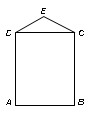# Problem Solving

The problem solving section is designed to test your problem solving skills, not your ability to perform such problems mathematically. Advanced math topics like trigonometry and calculus are not covered on the Test. So all you have to know to have a high score on this section is basic (high school level) arithmetic, geometry and algebra. A Quantitative refresher is sufficient to have mastery on this section.

### Major Topics Covered

Simple Arithematic, Simple Algebra, Averages, Ratios & Proportions, Percentage, Sets, Work problems, Speed and Motion, Combinations, Propability, and Geometry are basic topics covered on the test. You need not to go in details of mathematics including Calculus, Trignometery, Binomial theorem, Conics, and so on.

The important item you must practice is simple calculations. If you spend more time on simple calculatins during the test then you'll not be able to spend time on the undersatnding of the questions.

### Sample Questions

#### Question: 1

At the Luxury Ornament store, Nancy bought two tops for \$299 each and a ring for \$140. She paid 1/6 of total cost at the time of purchase and the balance in 12 equal monthly instalments. What was the amount of each month’s payment?
1. \$10.25
2. \$37.50
3. \$42.75
4. \$51.25
5. \$61.50

#### Question: 2

If f(x)= x2 - 3, what is the value of f(4)?
1. 16
2. 13
3. 19
4. 7
5. 0

#### Question 3In the figure, the area of square ABCD is 100, and the area of isosceles triangle DEC is 10. What is the distance from A to E?
1. 16
2. 13
3. 19
4. 7
5. 0
• 1 : E
• 2 : B
• 3 : B

### SAT, GRE, GMAT, GAT

College of Admission Tests offers highly effective test preparation . Visit:

#### Merit and Waiting Lists of Pak Universities